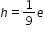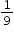# MA.7.AR.4.4

Given any representation of a proportional relationship, translate the representation to a written description, table or equation.

### Examples

Example: The written description, there are 60 minutes in 1 hour, can be represented as the equation m=60h.

Example: Gina works as a babysitter and earns \$9 per hour. She would like to earn \$100 to buy a new tennis racket. Gina wants to know how many hours she needs to work. She can use the equation, where e is the amount of money earned, h is the number of hours worked andis the constant of proportionality.

### Clarifications

Clarification 1: Given representations are limited to a written description, graph, table or equation.

Clarification 2: Instruction includes equations of proportional relationships in the form of y=px, where p is the constant of proportionality.

General Information
Subject Area: Mathematics (B.E.S.T.)
Strand: Algebraic Reasoning
Status: State Board Approved

## Related Courses

This benchmark is part of these courses.
1205040: M/J Grade 7 Mathematics (Specifically in versions: 2014 - 2015, 2015 - 2022, 2022 and beyond (current))
1205050: M/J Accelerated Mathematics Grade 7 (Specifically in versions: 2014 - 2015, 2015 - 2020, 2020 - 2022, 2022 and beyond (current))
1204000: M/J Foundational Skills in Mathematics 6-8 (Specifically in versions: 2014 - 2015, 2015 - 2022, 2022 and beyond (current))
7812020: Access M/J Grade 7 Mathematics (Specifically in versions: 2014 - 2015, 2015 - 2018, 2018 - 2019, 2019 - 2022, 2022 and beyond (current))

## Related Access Points

Alternate version of this benchmark for students with significant cognitive disabilities.
MA.7.AR.4.AP.4: Given a table representation of a proportional relationship, translate the relationship into an equation or a graph.

## Related Resources

Vetted resources educators can use to teach the concepts and skills in this benchmark.

## Formative Assessments

Writing An Equation:

Students are asked to write an equation to represent a proportional relationship depicted in a graph.

Type: Formative Assessment

Serving Size:

Students are given the number of calories in a serving of oatmeal and are asked to write an equation that models the relationship between the size of the serving and the number of calories.

Type: Formative Assessment

## Lesson Plan

Cricket Songs:

Using a guided-inquiry model, students in a math or science class will use an experiment testing the effect of temperature on cricket chirping frequency to teach the concepts of representative vs random sampling, identifying directly proportional relationships, and highlight the differences between scientific theory and scientific law.

Type: Lesson Plan

## MFAS Formative Assessments

Serving Size:

Students are given the number of calories in a serving of oatmeal and are asked to write an equation that models the relationship between the size of the serving and the number of calories.

Writing An Equation:

Students are asked to write an equation to represent a proportional relationship depicted in a graph.

## Student Resources

Vetted resources students can use to learn the concepts and skills in this benchmark.

## Parent Resources

Vetted resources caregivers can use to help students learn the concepts and skills in this benchmark.